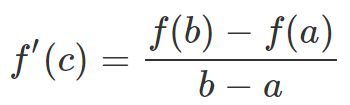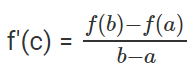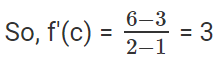Courses

# Mean Value Theorem JEE Notes | EduRev

## JEE : Mean Value Theorem JEE Notes | EduRev

The document Mean Value Theorem JEE Notes | EduRev is a part of the JEE Course Mathematics For JEE.
All you need of JEE at this link: JEE

In mathematics, the mean value theorem states, roughly, that given a planar arc between two endpoints, there is at least one point at which the tangent to the arc is parallel to the secant through its endpoints.
The Mean Value Theorem states that if f(x) is continuous on [a, b] and differentiable on (a, b) then there exists a number c between a and b such that:Solved Example

Question: Evaluate f(x) = x+ 2 in the interval [1, 2] using mean value theorem.
Solution:
Given function is:
f(x) = x+ 2
Interval is [1, 2].
i.e. a = 1, b = 2
Mean value theorem is given by,f(b) = f(2) = 22 + 2 = 6
f(a) = f(1) = 12 + 2 = 3Offer running on EduRev: Apply code STAYHOME200 to get INR 200 off on our premium plan EduRev Infinity!

## Mathematics For JEE

123 videos|273 docs|299 tests

,

,

,

,

,

,

,

,

,

,

,

,

,

,

,

,

,

,

,

,

,

;